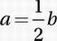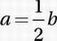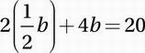# SAT Math Multiple Choice Question 721: Answer and Explanation

### Test Information

Question: 721

1. Ifand 2a + 4b = 20, what is the value of b?

• A. 2.5
• B. 4
• C. 5
• D. 15

Explanation:

B

Algebra (systems) EASY

Since the question asks for the value of b, it makes sense to substitute for a so that we get a single equation in terms of b.

Second equation:

2a + 4b = 20

SubstituteSimplify and combine:

b + 4b = 5b = 20

Divide by 5:

b = 4